# Electrical Permittivity by A.K.Sinha

Electrical Permittivity of the medium :

Electrical Permittivity of a medium is defined as the electeical characteristic of the medium due to which it absorbs some effect of electric force between two charges.

Simply you can understand it, as how a medium can influence the effect of electric force between two charges. It is denoted by ε ( Epsilon). The value of ε is different for different mediums.

To understand it better, let us consider a system of two point charges Q1 & Q2 placed R  m apart with a medium in between them.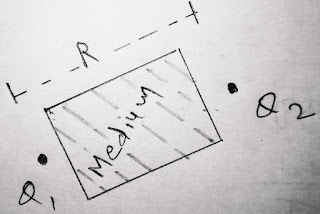Fig : 1

In this case the force of interaction between two charge is lowered due to presence of this medium in comparison with the situation shown in fig 2 , where two charges were considered in space or in air ( Fig 2).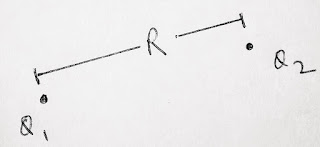Fig : 2

We know that according to coulomb's law for air or space , the force of interaction between two charges is given by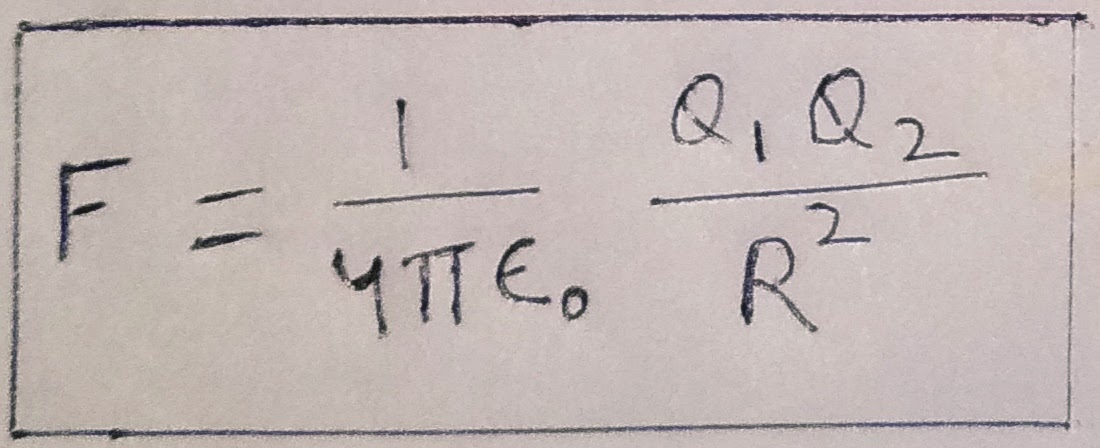..…..(1)

But if there is a medium m in between two charges then the form of above equation becomes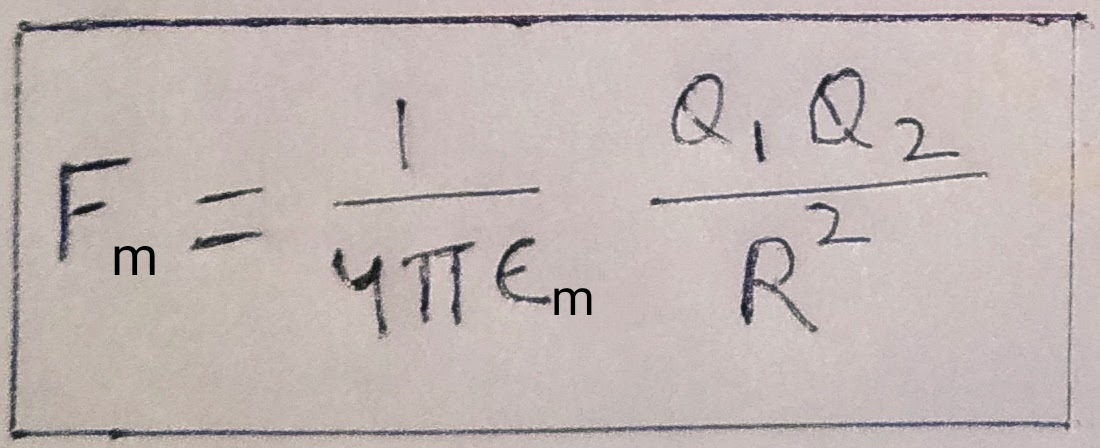……..( 2)

Here εm is absolute electrical Permittivity of the given medium. If the medium in between two charges is air then we simply use εm = εo  ( i.e. absolute Permittivity of free space) in the above equation 2 and we get the equation 1 for air or space as a medium.

From above equation we can conclude that the force of interaction between two charges depends inversely on Permittivity of medium as if the Permittivity of the medium is large then the force of interaction between two charges get lowered and vice versa.

Dielectric Constant or Relative Permittivity :

Dielectric constant or Relative Permittivity  of a medium is defined as the ratio of absolute electrical Permittivity of the medium to the absolute electrical Permittivity of the air or space. It is denoted by εr or K .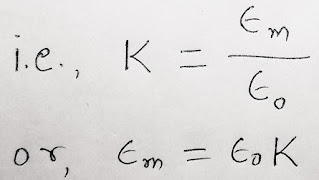Using above value of εm in equation (2), we can rewrite the coulomb's inverse square law into a more simplified form as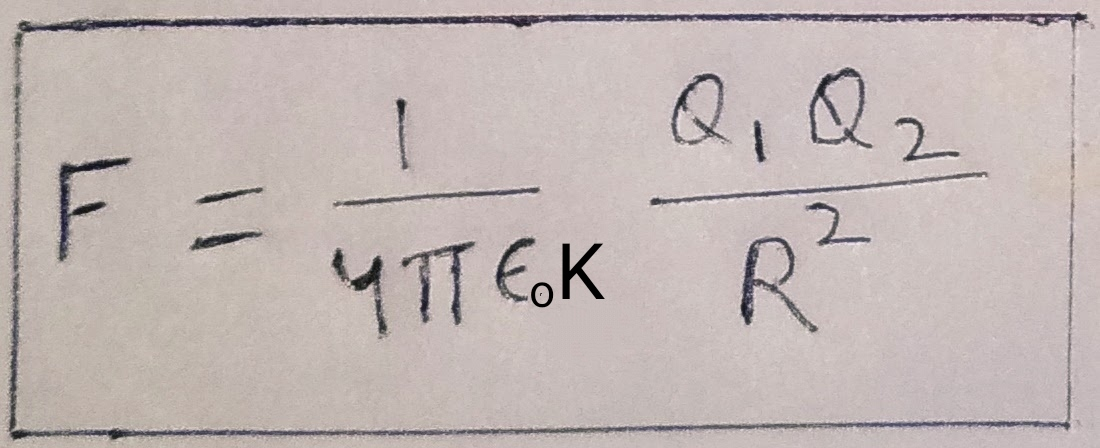……….(3)

( NOTE : This is the more useful form of inverse square law in numericals for higher secondary level and Diploma level students.